# Net (of sets in a topological space)

(diff) ← Older revision | Latest revision (diff) | Newer revision → (diff)
A family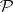of subsets of a topological spacesuch that for eachand each neighbourhoodofthere is an elementofsuch that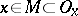.
The family of all one-point subsets of a space and every base of a space are networks. The difference between a network and a base is that the elements of a network need not be open sets. Networks appear under continuous mappings: Ifis a continuous mapping of a topological spaceonto a topological spaceand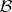is a base of, then the images of the elements ofunderform a networkin. Further, ifis covered by a family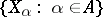of subspaces, then, taking for each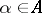any base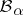ofand amalgamating these bases, a network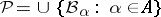inis obtained. Spaces with a countable network are characterized as images of separable metric spaces under continuous mappings.
The minimum cardinality of a network of a spaceis called the network weight, or net weight, ofand is denoted by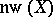. The net weight of a space never exceeds its weight (cf. Weight of a topological space), but, as is shown by the example of a countable space without a countable base, the net weight can differ from the weight. For compact Hausdorff spaces the net weight coincides with the weight. This result extends to locally compact spaces, Čech-complete spaces and feathered spaces (cf. Feathered space). Hence, in particular, it follows that weight does not increase under surjective mappings of such spaces. Another corollary: If a feathered space(in particular, a Hausdorff compactum) is given as the union of a family of cardinality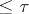of subspaces, the weight of each of which does not exceed, supposed infinite, then the weight ofdoes not exceed.